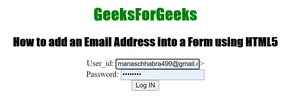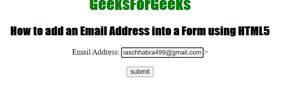Open in App
Not now

# How to Insert Email Address into Form using HTML5 ?

• Last Updated : 27 Sep, 2021

In this article, we will learn how to add and get an email address of a user as data of the form by adding an email input field. As we know that an email_id is a vital component of user data. Email address is used for the verification of the user. It is also used to make contact with the submitters directly.

Approach: To complete this task, we have to follow the below steps-

• Create an HTML document that contains an <input> tag.
• Use the type attribute with the <input> element which is set to value “email”.

Syntax

`<input type="email">`

Example 1: In this example, we are creating a form containing email id (username) and password.

## HTML

 ```<``html``>` `<``head``>``    ``<``title``>``        ``How to add an Email Address``        ``into a Form using HTML5?``    ``` `    ``<``style``>``        ``#Geek_p {``            ``font-size: 30px;``            ``color: green;``        ``}` `        ``h1,``        ``h2 {``            ``font-family: impact;``        ``}``    ````` `<``body` `style``=``"text-align: center"``>``    ``<``h1` `style``=``"color: green"``>GeeksForGeeks` `    ``<``h2``>``        ``How to add an Email Address``        ``into a Form using HTML5``    ````    ``<``form``>``        ``User_id:``        ``<``input` `type``=``"email"``>>``        ``<``br` `/>` `        ``Address:``        ``<``input` `type``=``"password"``>``        ``<``br` `/>``        ` `        ``<``button``>Log IN``    ````` ``

Output:Example 2: In this example, we are creating an input field of type email.

## HTML

 ```<``html``>` `<``head``>``    ``<``title``>``        ``How to add an Email Address``        ``into a Form using HTML5?``    ``` `    ``<``style``>``        ``#Geek_p {``            ``font-size: 30px;``            ``color: green;``        ``}` `        ``h1,``        ``h2 {``            ``font-family: impact;``        ``}``    ````` `<``body` `style``=``"text-align: center"``>``    ``<``h1` `style``=``"color: green"``>GeeksForGeeks` `    ``<``h2``>``        ``How to add an Email Address``        ``into a Form using HTML5``    ````    ``<``form``>``        ``Email Address:``        ``<``input` `type``=``"email"``>>``        ``<``br` `/>``        ``<``br` `/>``        ` `        ``<``button``>submit``    ````` ``

Output:My Personal Notes arrow_drop_up